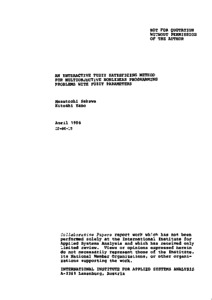# An Interactive Fuzzy Satisficing Method for Multiobjective Nonlinear Programming Problems with Fuzzy Parameters

Sakawa, M. & Yano, H. (1986). An Interactive Fuzzy Satisficing Method for Multiobjective Nonlinear Programming Problems with Fuzzy Parameters. IIASA Collaborative Paper. IIASA, Laxenburg, Austria: CP-86-015Preview
Text
CP-86-015.pdf

## Abstract

This paper presents an interactive fuzzy satisficing method for multiobjective nonlinear programming problems with fuzzy parameters. The fuzzy parameters in the objective functions and the constraints are characterized by the fuzzy numbers. On the basis of the alpha-level sets of the fuzzy numbers, the concept of alpha-multiobjective nonlinear programming and alpha-Pareto optimality is introduced. Through the interaction with the decision maker (DM), the fuzzy goals of the DM for each of the objective functions in alpha-multiobjective nonlinear programming are quantified by eliciting the corresponding membership functions. After determining the membership functions, in order to generate a candidate for the satisficing solution which is also alpha-Pareto optimal, if the DM specifies the degree alpha of the alpha-level sets and the reference membership values, the augmented minimax problem is solved and the DM is supplied with the corresponding alpha-Pareto optimal solution together with the trade-off rates among the values of the membership functions and the degree alpha. Then by considering the current values of the membership functions and as well as the trade-off rates, the DM responds by updating his reference membership values and/or the degree alpha. In this way the satisficing solution for the DM can be derived efficiently from among an alpha-Pareto optimal solution set. Based on the proposed method, a time-sharing computer program is written and an illustrative numerical example is demonstrated along with the corresponding computer outputs.

Item Type: Monograph (IIASA Collaborative Paper) System and Decision Sciences - Core (SDS) IIASA Import 15 Jan 2016 01:57 27 Aug 2021 17:12 http://pure.iiasa.ac.at/2870View Item